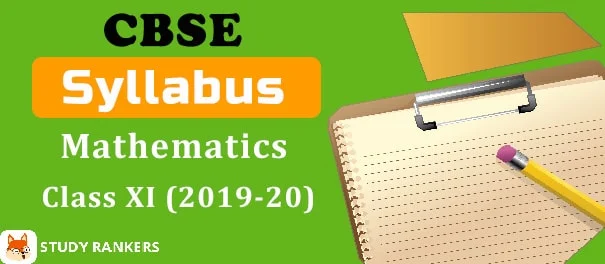## CBSE Class 11 Mathematics Syllabus 2019-20

CBSE has released the Class 11 Mathematics Syllabus 2019-20. The annual board examination will be of 80 marks, with a duration of three hours. There will be internal assessment (Periodic Tests and Mathematics Activities) for 20 Marks.In Board examination, the entire syllabus will come. The written examination will be of 80 marks. No chapter/unit-wise weightage is given.

 No. Units Marks I Sets and Functions 23 II Algebra 30 III Coordinate Geometry 10 IV Calculus 05 V Mathematical Reasoning 02 VI Statistics and Probability 10 - Total 80 - Internal Assessment 20

Unit-I: Sets and Functions

• Sets
• Relations and Functions
• Trigonometric Functions

Unit-II: Algebra

• Principle of Mathematical Induction
• Complex Numbers and Quadratic Equations
• Linear Inequalities
• Permutations and Combinations
• Binomial Theorem
• Sequence and Series

Unit-III: Coordinate Geometry

• Straight Lines
• Conic Sections
• Introduction to Three-dimensional Geometry

Unit-IV: Calculus

• Limits and Derivatives

Unit-V: Mathematical Reasoning

• Mathematical Reasoning

Unit-VI: Statistics and Probability

• Statistics
• Probability

Question Wise Breakup

 Type of Question Mark per Question Total No. of Questions Total Marks VSA 1 20 20 SA 2 6 12 LA I 4 6 24 LA II 6 4 24 Total - 36 80

Prescribed Books:

1) Mathematics Textbook for Class XI, NCERT Publications